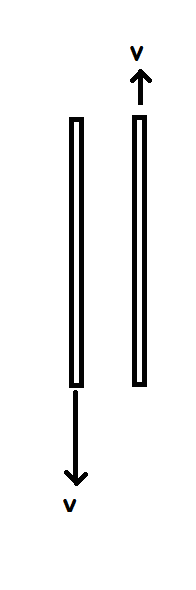# Force of interaction between fast moving capacitor plates.The plates of a square plate capacitor are separated by a very small distance and have charge $q = 1 \mu C$ side length $l = 1m$ suddenly start moving in opposite directions with equal speed $v = 2 \times 10^8 m/s$ as shown. Find the force experienced by one plate due to the other at this instant in Newtons.

×